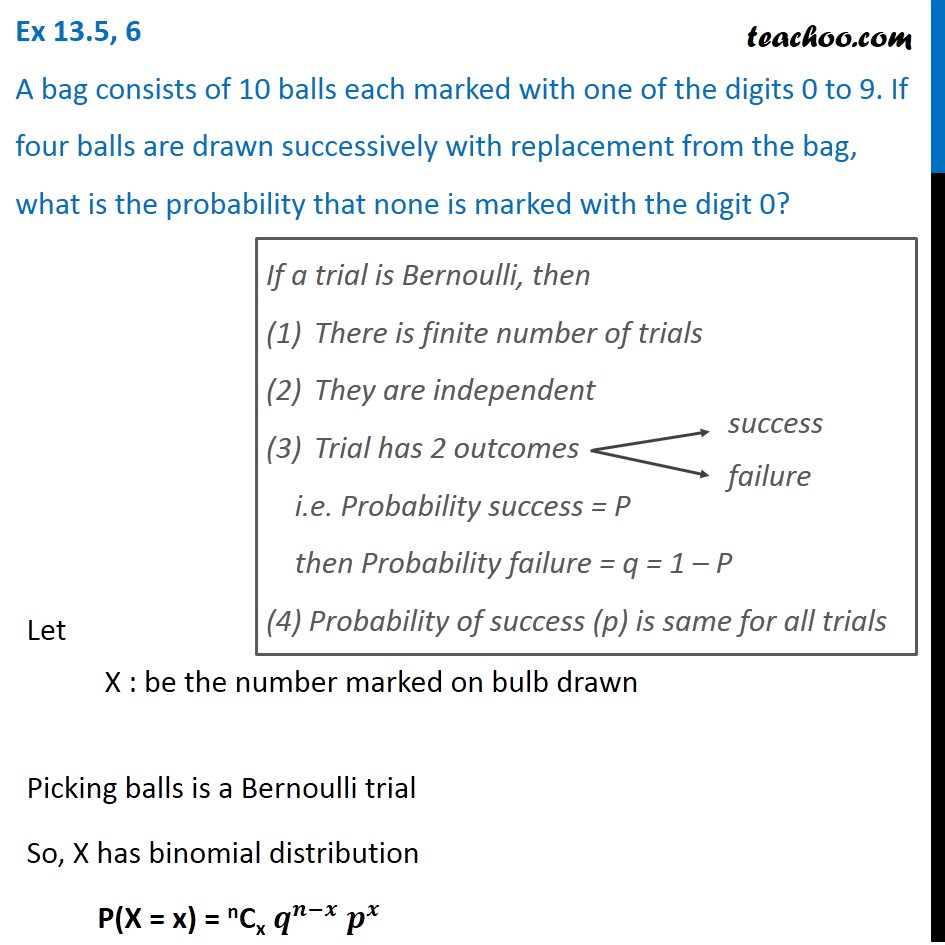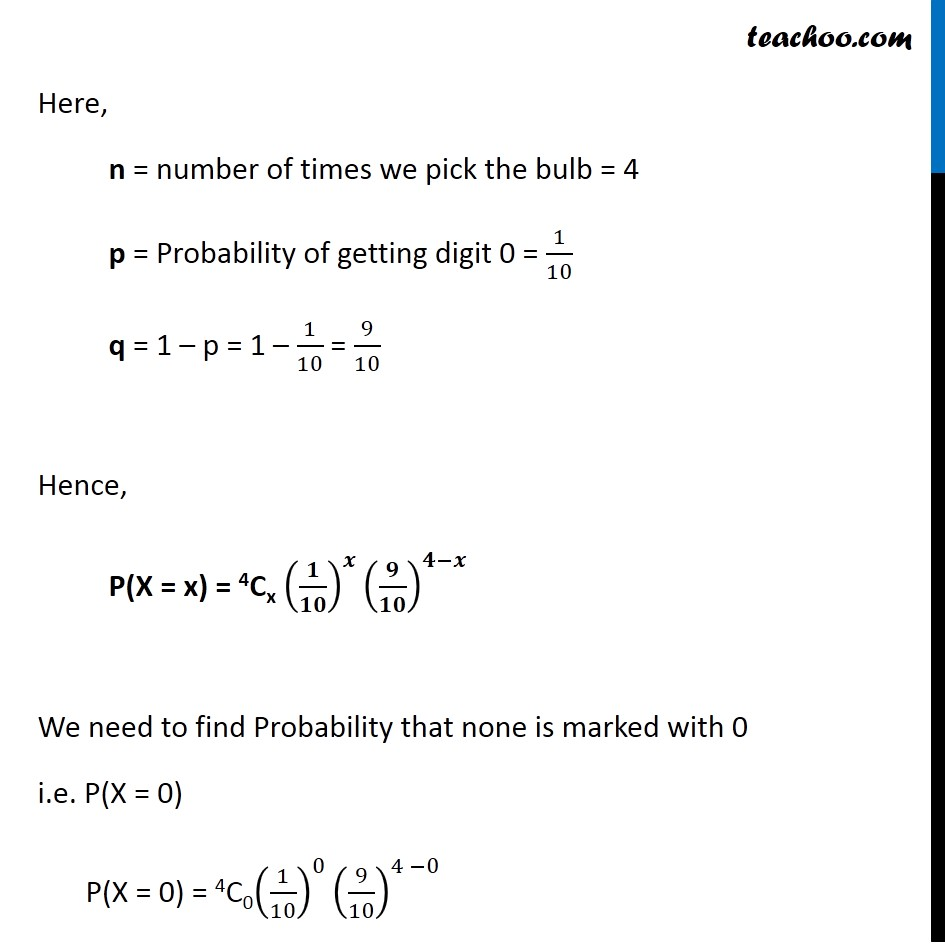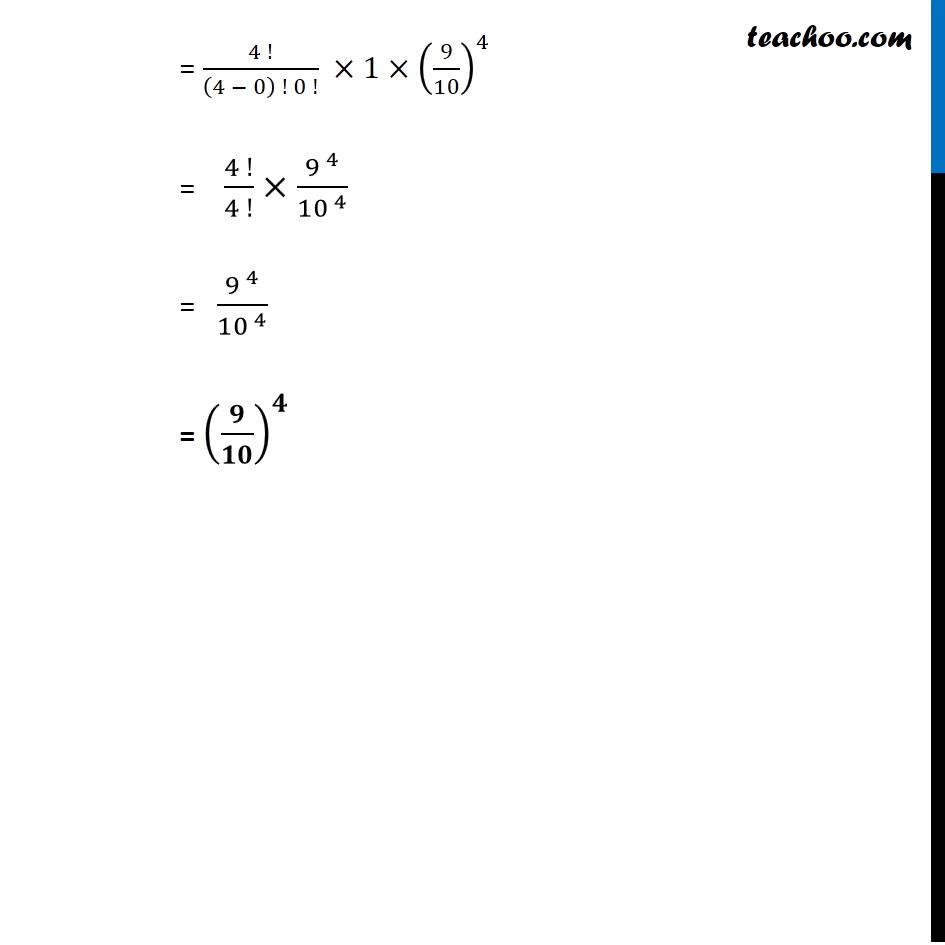Chapter 13 Class 12 Probability

Class 12
Important Questions for exams Class 12Learn in your speed, with individual attention - Teachoo Maths 1-on-1 Class

### Transcript

Question 6 A bag consists of 10 balls each marked with one of the digits 0 to 9. If four balls are drawn successively with replacement from the bag, what is the probability that none is marked with the digit 0?If a trial is Bernoulli, then There is finite number of trials They are independent Trial has 2 outcomes i.e. Probability success = P then Probability failure = q = 1 – P (4) Probability of success (p) is same for all trials Let X : be the number marked on bulb drawn Picking balls is a Bernoulli trial So, X has binomial distribution P(X = x) = nCx 𝒒^(𝒏−𝒙) 𝒑^𝒙 Here, n = number of times we pick the bulb = 4 p = Probability of getting digit 0 = 1/10 q = 1 – p = 1 – 1/10 = 9/10 Hence, P(X = x) = 4Cx (𝟏/𝟏𝟎)^𝒙 (𝟗/𝟏𝟎)^(𝟒−𝒙) We need to find Probability that none is marked with 0 i.e. P(X = 0) P(X = 0) = 4C0(1/10)^0 (9/10)^(4 −0) = (4 !)/((4 − 0) ! 0 !) ×1×(9/10)^4 = (4 !)/(4 !)×〖9 〗^4/〖10〗^( 4) = 〖9 〗^4/〖10〗^( 4) = (𝟗/𝟏𝟎)^𝟒